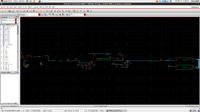# [Moved]: discrete time integrator with transfer function = 1/(1-Z^-1)

1. ## [Moved]: discrete time integrator with transfer function = 1/(1-Z^-1)

Hi,
I am designing a discrete time integrator with transfer function = 1/(1-Z^-1) using model writer of the cadence virtuoso, below is the verilogA code of the integrator. But I am getting a gain of more than 1000 in the output, when applying an input signal of 900 uV (p-p).

I added the screenshot of the schematic as well as the transient output response of the integrator.```Code Verilog - [expand]1
2
3
4
5
6
7
8
9
10
11
12
13
14
15
16
17
18
19
20
21
22
23
24
25
26
27
28
29
30
31
32
33
34
35
36
37
38
39
40
41
42
43
44
45
46
47
48
49
50
51
52
53
// FUNCTION: Z-domain Filter
// VERSION: \$Revision: 2.8 \$
// AUTHOR: Cadence Design Systems, Inc.
//
// GENERATED BY: Cadence Modelwriter 2.31
// ON: Thu Feb 02 08:59:10 IST 2017
//
// Description: N degree Transfer functions of H(z)
// May be specified as a Polynomial or as Poles and Zeros.
//
// Complex Poles and Zeros must have conjugates.
//
//
// This model is an example, provided "as is" without express or
// implied warranty and with no claim as to its suitability for
// any purpose.
//
// PARAMETERS:
// first = Delay before taking first sample [S]
// samp = Sampling period [S]
// trans = Transtion time [S]
//

`include "discipline.h"
`include "constants.h"

// model ztrans

// Z-domain in Numerator-Denominator form
// Filter order is: 1 / 2

module ztrans (vin, vout);
input vin;
output vout;
electrical vin, vout;
parameter real samp = 3.90u from (0:inf);

// nxN is numerator of degree N.
// dxN is denominator of degree N.
parameter real nx0 = 1.0;
parameter real dx0 = 1.0;
parameter real dx1 = -1;

analog begin
V(vout) <+ zi_nd( V(vin), { nx0 },
{ dx0, dx1 }, samp);
end

endmodule```•

2. ## Re: discrete time integrator with transfer function = 1/(1-Z^-1)

I see a gain of about 50 in the waveforms which should be expected for the given parameters. (T=3.9 µs, f = 1 kHz).

1 members found this post helpful.3. ## Re: discrete time integrator with transfer function = 1/(1-Z^-1)

Thanks FvM for the reply,
First I apologize for typing gain = 1000.
1. But this is an ideal delay free integrator isn't it suppose to give gain = 1/sqrt(2) only.
2. when I am giving input 200 mV (peak-peak) then the output is in kVs.
3. I am simulating in cadence virtuoso IC 6.1.5, so is there any settings required to converge the output, as I think the output of the ideal delay free integrator is not converging.
Please correct me where I am wrong.•

4. ## Re: discrete time integrator with transfer function = 1/(1-Z^-1)

1. Why 1/sqrt(2)? It's not time continuous integral with time constant 1s, it's time discrete integral with 3.9µs sampling interval, roughly equivalent to 3.9 µs integrator time constant. Gain for sine input is 1/(ω*3.9µs).

3. Ideal integrator output never converges, neither continuous nor time discrete. External feedback or at least initial conditions needed.

1 members found this post helpful.5. ## Re: discrete time integrator with transfer function = 1/(1-Z^-1)

Still there exist many bugs in Z-domain function of Verilog-A.
So I don't recommend use Z-domain function of Verilog-A.

Use "pllMMLib/z_integrator_digi".

1 members found this post helpful.6. ## Re: discrete time integrator with transfer function = 1/(1-Z^-1)

An integrator is just that - it takes the existing sample, scales it and accumulates the result. It will happily count towards infinity (infinite gain) if the input stays positive or negative for a long time (I.E. low frequency AC or DC)

I wonder if an ideal integrator is really what you want or if you want the equivalent of an RC filter which is slightly different (has capped DC gain, often 1).

1 members found this post helpful.•

7. thanks for the reply FvM.

Thanks for the reply pancho_hideboo, but What is "pIIMMLib" which library is this??

Thanks for the reply asdf44,
Please see the image attached with this reply,http://obrazki.elektroda.pl/9756054400_1486740617.png

This is a second order sigma-delta modulator (all blocks are ideal verilogA models only) schematic design, problems with this is:
1. Although there is a negative feedback, the output of the integrator is very high in kVs due to which the ADC (4-bits) always give logic 1 and corresponding the DAC (4-bits) output is Vmax (= 1.8 V in this case).
2. If we use all the blocks of the schematic except the integrator the system works perfectly fine (i.e ADC, DAC, Sample&Hold and vcvs blocks work fine).
3. The input signal is 200 mV (peak-peak) and frequency = 22 kHz, while the sampling clock is operating at 2.8 MHz.8. ## Re: [moved] discrete time integrator with transfer function = 1/(1-Z^-1)Originally Posted by Vivek_2017Thanks for the reply pancho_hideboo, but What is "pIIMMLib" which library is this??
"pllMMLib" is located in same directory as "rfLib", "bmslib", "pllLib" and "ahdlLib" which are shipped with Cadence dfII.

"Your_CDS_Install_Directory"/tools/dfII/samples/artistOriginally Posted by Vivek_20172. If we use all the blocks of the schematic except the integrator the system works perfectly fine (i.e ADC, DAC, Sample&Hold and vcvs blocks work fine).
Don't use zi_nd() of Verilog-A.--[[ ]]--